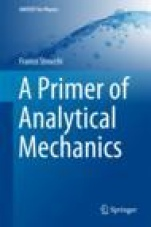### Logowanie

Zapomniałeś hasła?
Nowy klient

Jeżeli nie znalazłeś poszukiwanej książki, skontaktuj się z nami wypełniając formularz kontaktowy.

Ta strona używa plików cookies, by ułatwić korzystanie z serwisu. Mogą Państwo określić warunki przechowywania lub dostępu do plików cookies w swojej przeglądarce zgodnie z polityką prywatności.

### Informacje szczegółowe o książce# A Primer of Analytical Mechanics

##### ISBN 9783319737607

Autor: Franco Strocchi

Wydawca: Springer

Dostępność: Dostawa 10-20 dni

Cena: 162,75 zł

 ISBN13: 9783319737607 Autor: Franco Strocchi Oprawa: Hardback Rok Wydania: 2018 Ilość stron: 114

This book presents the basic elements of Analytical Mechanics, starting from the physical motivations that favor it with respect to the Newtonian Mechanics in Cartesian coordinates. Rather than presenting Analytical Mechanics mainly as a formal development of Newtonian Mechanics, it highlights its effectiveness due to the following five important achievements: 1) the most economical description of time evolution in terms of the minimal set of coordinates, so that there are no constraint forces in their evolution equations; 2) the form invariance of the evolution equations, which automatically solves the problem of fictitious forces; 3) only one scalar function encodes the formulation of the dynamics, rather than the full set of vectors which describe the forces in Cartesian Newtonian Mechanics; 4) in the Hamiltonian formulation, the corresponding evolution equations are of first order in time and are fully governed by the Hamiltonian function (usually corresponding to the energy); 5) the emergence of the Hamiltonian canonical algebra and its effectiveness in simplifying the control of the dynamical problem (e.g. the constant of motions identified by the Poisson brackets with the Hamiltonian, the relation between symmetries and conservations laws, the use of canonical transformations to reduce the Hamiltonian to a simpler form etc.). The book also addresses a number of points usually not included in textbook presentations of Analytical Mechanics, such as 1) the characterization of the cases in which the Hamiltonian differs from the energy, 2) the characterization of the non-uniqueness of the Lagrangian and of the Hamiltonian and its relation to a ?gauge? transformation, 3) the Hamiltonian formulation of the Noether theorem, with the possibility that the constant of motion corresponding to a continuous symmetry of the dynamics is not the canonical generator of the symmetry transformation but also involves the generator of a gauge transformation. In turn, the book?s closing chapter is devoted to explaining the extraordinary analogy between the canonical structure of Classical and Quantum Mechanics. By correcting the Dirac proposal for such an explanation, it demonstrates that there is a common Poisson algebra shared by Classical and Quantum Mechanics, the differences between the two theories being reducible to the value of the central variable of that algebra.

Preface.- 1 Difficulties of Cartesian Newtonian Mechanics.- 1.1 Constraint forces.- 1.2 Non-inertial frames and fictitious forces.- 2 Lagrange equations.- 2.1 Degrees of freedom and Lagrangian coordinates.- 2.2 Lagrangian form of Newton's equations.- 2.3 Lagrange equations.- 2.4 Lagrange equations at work. Examples.- 2.5 Generalized potential.- 2.6 Larmor theorem.- 2.7 Physical meaning of Lagrange equations; conjugate momenta.- 2.8 Cyclic variables, symmetries and conserved conjugate momenta.- 2.9 Non-uniqueness of the Lagrangian.- 3 Hamilton equations.- 3.1 Energy conservation.- 3.2 Hamilton equations.- 3.3 Coordinate transformations and Hamilton equations.- 3.4 Canonical transformations.- 4 Poisson brackets and canonical structure.- 4.1 Constants of motion identified by.- Poisson brackets.- 4.2 General properties of Poisson brackets.- 4.3 Canonical structure.- 4.4 Invariance of Poisson brackets under canonical transformations.- 5 Generation of canonical transformations.- 5.1 Alternative characterization of canonical transformations.- 5.2 Extended canonical transformations.- 5.3 Generators of continuous groups of canonical transformations.- 5.4 Symmetries and conservation laws. Noether theorem.- 6 Small oscillations.- 6.1 Equilibrium configurations. Stability.- 6.2 Small oscillations.- 7 The common Poisson algebra of classical and quantum mechanics.- 7.1 Dirac Poisson algebra.- 7.2 A common Poisson algebra of classical and quantum mechanics.- Index.

### Koszyk

Książek w koszyku: 0 szt.

Wartość zakupów: 0,00 zł

### Kontakt

Gambit
Centrum Oprogramowania
i Szkoleń Sp. z o.o.

Al. Pokoju 29b/22-24

31-564 Kraków

+48 12 414 3791

+48 12 414 3387

Siedziba Księgarni

ul. Kordylewskiego 1

31-542 Kraków

+48 12 410 5989

+48 12 414 3767

Wyślij e-mail

### Subskrypcje

Administratorem danych osobowych jest firma Gambit COiS Sp. z o.o. Na podany adres będzie wysyłany wyłącznie biuletyn informacyjny.

### Autoryzacja płatności